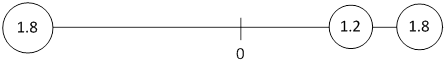### Sample ProblemThree masses are attached to a weightless pole. The first mass is 1.8 kg and positioned 2.0 meters to the left of the center of the pole. The second mass is 1.2 kg and is placed .80 meters to the right of the center. The last mass is 1.8 kg and is placed 1.0 meters to the right of the center.

Where is the center of mass of the pole? Select all the correct answers.

.18 meters to the left

.18 meters to the right

2 meters to the left

-.18 meters to the right

-2 meters to the right

-2 meters to the left

#### Solution

We use the formula x = (m1 x1 + m2 x2 + … + mn xn) / (m1 + m2 + … + mn) , and we are given m1 = 1.8, m2 = 1.2, m3 = 1.8, and x1 = -2.0, x2 = .80, x3 = 1.0. We plug all of these values into the equation to get that x = -.18 meters to the left of the center